# Merge DataFrames by Column Names in R

• Last Updated : 14 Sep, 2021

In this article, we are going to see how to merge Dataframe by Column Names using merge in R Programming Language.

The merge() function in base R can be used to merge input dataframes by common columns or row names. The merge() function retains all the row names of the dataframes, behaving similarly to the inner join. The dataframes are combined in order of the appearance in the input function call.

Syntax: merge(x, y, by, all)

Arguments :

• x, y – The input dataframes
• by – specifications of the columns used for merging. In case of merging using row names, the by attribute uses ‘row.names’ value.
• all – logical true or false.

### Example 1: Merge two dataframe by columns

Two dataframes can be merged together using the common columns, in both the dataframes. The column to use for merging can be specified in the “by” parameter during the function call. The output dataframe produces the rows equivalent to the common entries encountered in the columns specified in the “by” argument.

## R

 `# creating first dataframe``df1 <- ``data.frame``(col1 = ``LETTERS``[1:6],``                  ``col2a = ``c``(5:10),``                  ``col3a = ``TRUE``)`` ` `print ``(``"First DataFrame"``)``print ``(df1)``df2 <- ``data.frame``(col1 = ``LETTERS``[4:8],``                  ``col2b= ``c``(4:8),``                  ``col3b = ``FALSE``)`` ` `print ``(``"Second DataFrame"``)``print ``(df2)``df_merge <- ``merge``(df1,df2,by=``"col1"``)`` ` `print``(``"Merged DataFrame"``)``print ``(df_merge)`

Output: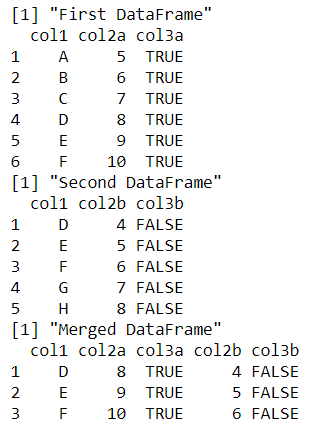### Example 2: Merge dataframe with missing values

In order to retain all the values of the first dataframe, irrespective of whether they have common values or not in the “by” parameter, we set all.x = true. The missing values belonging to the second dataframe are appended with a NA value.

## R

 `# creating first dataframe``df1 <- ``data.frame``(col1 = ``LETTERS``[1:6],``                  ``col2a = ``c``(5:10),``                  ``col3a = ``TRUE``)`` ` `print ``(``"First DataFrame"``)``print ``(df1)``df2 <- ``data.frame``(col1 = ``LETTERS``[4:8],``                  ``col2b= ``c``(4:8),``                  ``col3b = ``FALSE``)`` ` `print ``(``"Second DataFrame"``)``print ``(df2)``df_merge <- ``merge``(df1, df2, by = ``"col1"``,``                  ``all.x = ``TRUE``)`` ` `print``(``"Merged DataFrame"``)``print ``(df_merge)`

Output: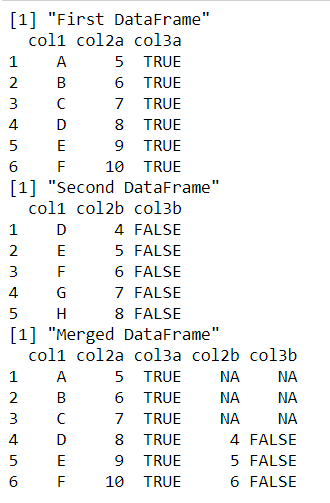In order to retain all the values of the second dataframe, irrespective of whether they have common values or not in the “by” parameter, we set all.y = true. The missing values belonging to the first dataframe columns are appended with a NA value.

## R

 `# creating first dataframe``df1 <- ``data.frame``(col1 = ``LETTERS``[1:6],``                  ``col2a = ``c``(5:10),``                  ``col3a = ``TRUE``)`` ` `print ``(``"First DataFrame"``)``print ``(df1)``df2 <- ``data.frame``(col1 = ``LETTERS``[4:8],``                  ``col2b= ``c``(4:8),``                  ``col3b = ``FALSE``)`` ` `print ``(``"Second DataFrame"``)``print ``(df2)``df_merge <- ``merge``(df1, df2, by = ``"col1"``, ``                  ``all.y = ``TRUE``)`` ` `print``(``"Merged DataFrame"``)``print ``(df_merge)`

Output: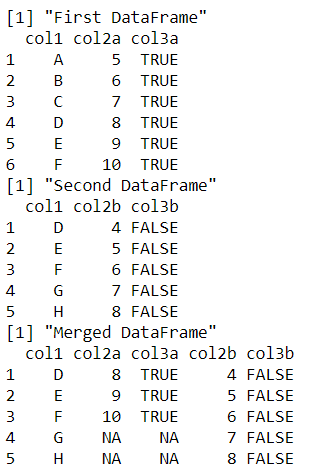The following code illustrates the usage when all the rows of both the input dataframes need to be retained.

## R

 `# creating first dataframe``df1 <- ``data.frame``(col1 = ``LETTERS``[1:6],``                  ``col2a = ``c``(5:10),``                  ``col3a = ``TRUE``)``print ``(``"First DataFrame"``)``print ``(df1)``df2 <- ``data.frame``(col1 = ``LETTERS``[4:8],``                  ``col2b= ``c``(4:8),``                  ``col3b = ``FALSE``)``print ``(``"Second DataFrame"``)``print ``(df2)``df_merge <- ``merge``(df1,df2,by=``"col1"``,all.x = ``TRUE``, all.y=``TRUE``)`` ` `print``(``"Merged DataFrame"``)``print ``(df_merge)`

Output: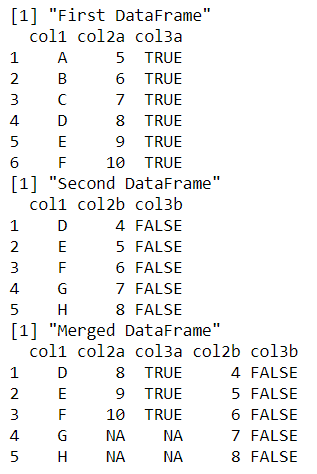### Example 3: Merge more than two dataframes

More than two dataframes can also be merged. However, the dataframes are merged using the merge() method call, two at a time in the order of their appearance in the function call. Therefore, if n dataframes are to be merged, n-1 function calls are required.

## R

 `# creating first dataframe``df1 <- ``data.frame``(col1 = ``LETTERS``[1:6],``                  ``col2a = ``c``(5:10),``                  ``col3a = ``TRUE``)``print ``(``"First DataFrame"``)``print ``(df1)``df2 <- ``data.frame``(col1 = ``LETTERS``[4:8],``                  ``col2b= ``c``(4:8),``                  ``col3b = ``FALSE``)``print ``(``"Second DataFrame"``)``print ``(df2)``df3 <- ``data.frame``(col1 = ``LETTERS``[7:9],``                  ``col2c= ``c``(2:4),``                  ``col3c = ``NA``)``print ``(``"Third DataFrame"``)``print ``(df3)``df_merge12 <- ``merge``(df1,df2,by=``"col1"``,all.x=``TRUE``, all.y = ``TRUE``)``df_merge <- ``merge``(df_merge12,df3,by=``"col1"``,all.x=``TRUE``, all.y = ``TRUE``)`` ` `print``(``"Merged DataFrame"``)``print ``(df_merge)`

Output: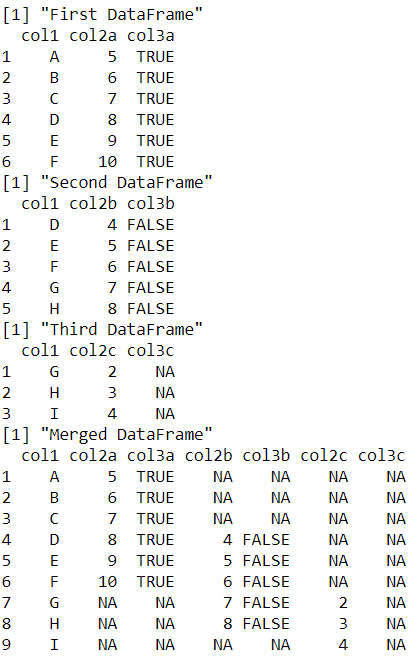My Personal Notes arrow_drop_up